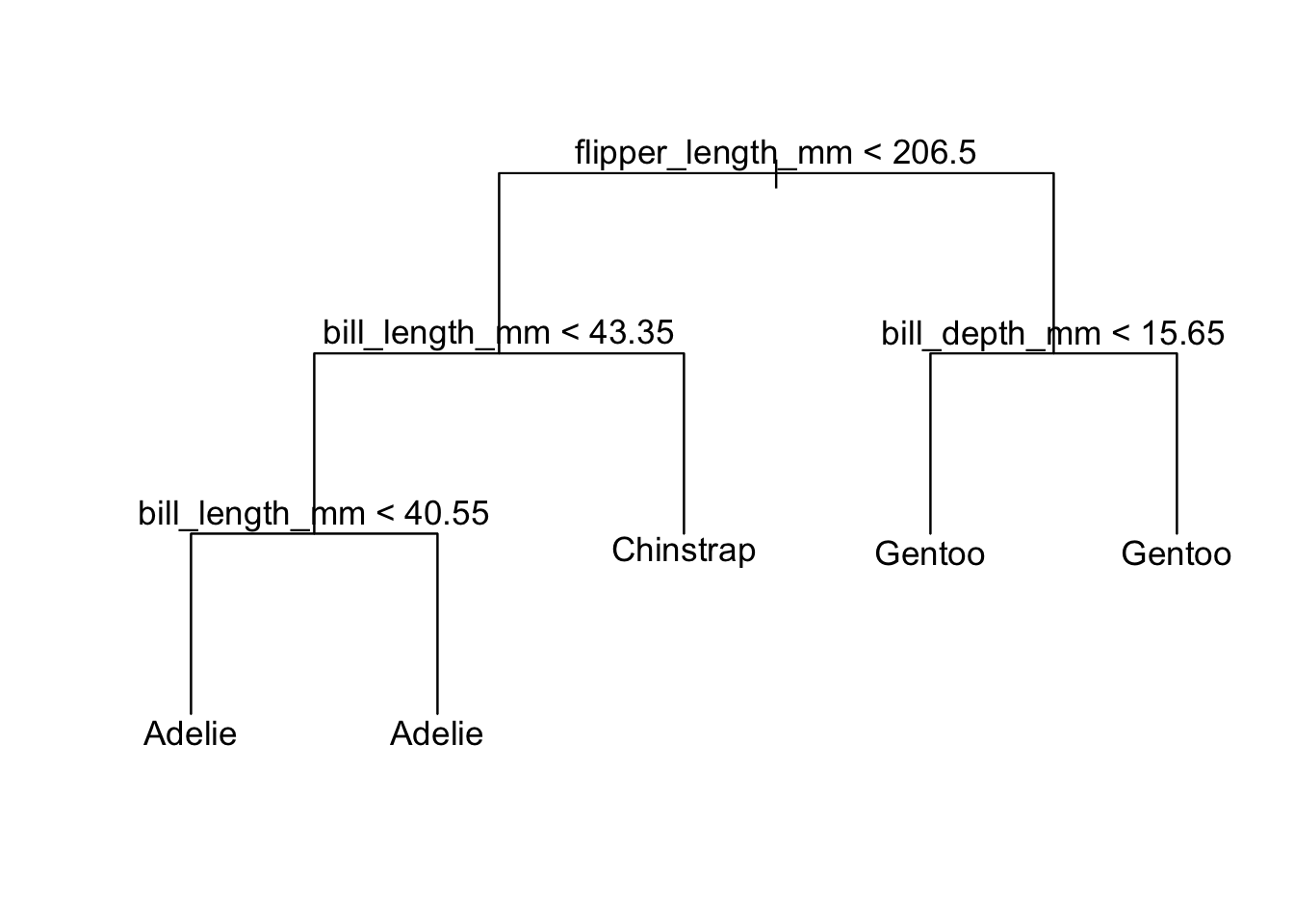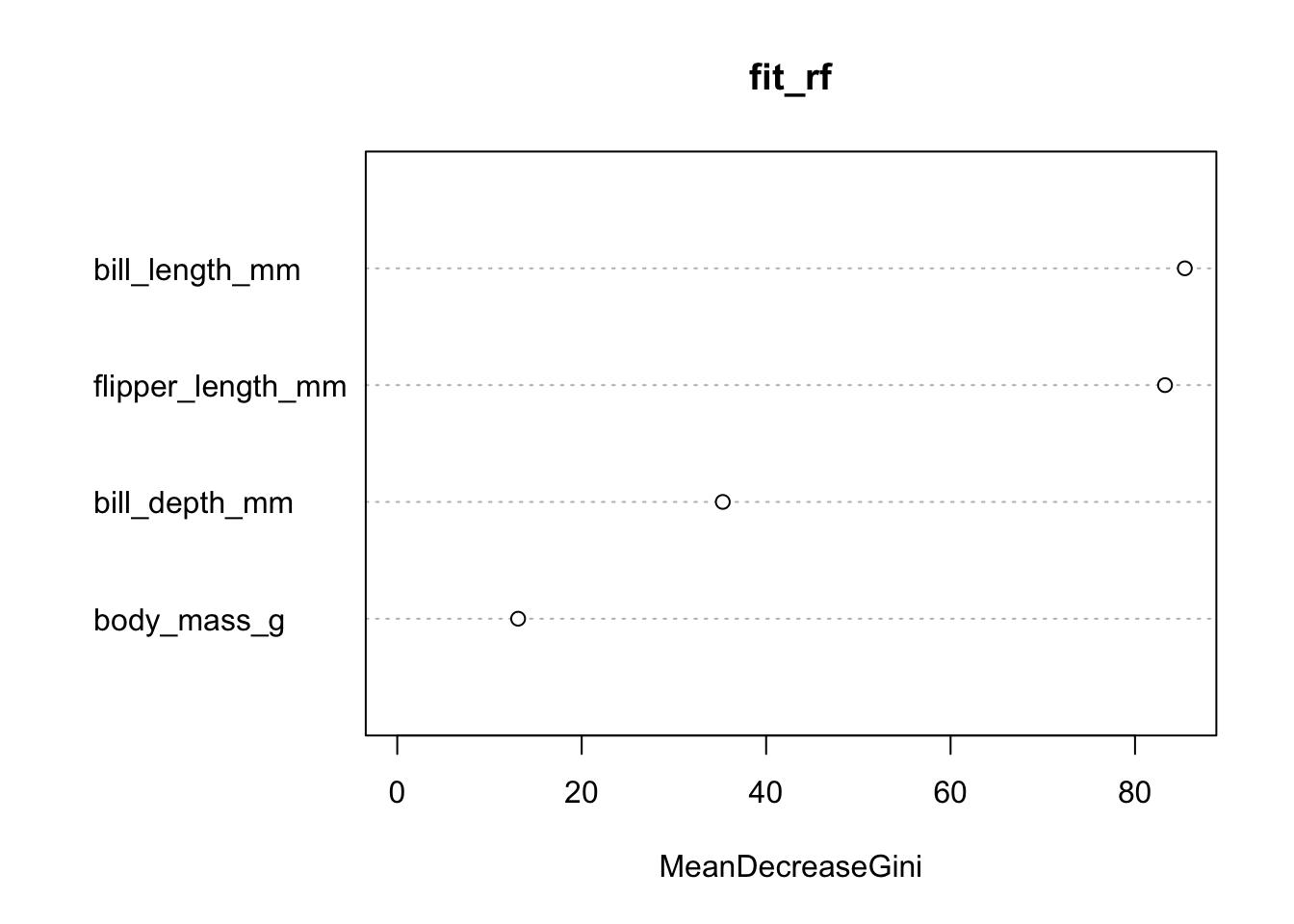## Random forest to classify penguins

``````pg <- penguins %>%
# not interested in classifying by time/island
dplyr::select(-island, -year, -sex) %>%
drop_na()``````

#### First fit a single tree

``````fit_tree <- tree(species ~ .,
split = "gini",
control = tree.control(nrow(pg), mincut = 40),
data = pg)
plot(fit_tree, type = "uniform")
text(fit_tree, pretty = 0, cex = 1.1)``````#### Fit a random forest

``````fit_rf <- randomForest(species ~ ., ntree = 100, mtry = 2,
minsize = 20, data = pg)
fit_rf``````
``````##
## Call:
##  randomForest(formula = species ~ ., data = pg, ntree = 100, mtry = 2,      minsize = 20)
##                Type of random forest: classification
##                      Number of trees: 100
## No. of variables tried at each split: 2
##
##         OOB estimate of  error rate: 2.92%
## Confusion matrix:
## Adelie       146         4      1 0.033112583
## Chinstrap      4        64      0 0.058823529
## Gentoo         0         1    122 0.008130081``````

#### Variable importance plot

Read `?importance` to learn more

``varImpPlot(fit_rf)``#### Partial dependence plots

``````pred_rf <- Predictor\$new(fit_rf)
pdp_rf <- FeatureEffects\$new(pred_rf,
features = c("bill_length_mm",
"flipper_length_mm"),
method = "pdp+ice")
plot(pdp_rf) ``````
``````## Warning: UNRELIABLE VALUE: Future ('future_lapply-1') unexpectedly generated
## random numbers without specifying argument 'future.seed'. There is a risk that
## those random numbers are not statistically sound and the overall results might
## be invalid. To fix this, specify 'future.seed=TRUE'. This ensures that proper,
## parallel-safe random numbers are produced via the L'Ecuyer-CMRG method. To
## disable this check, use 'future.seed=NULL', or set option 'future.rng.onMisuse'
## to "ignore".``````